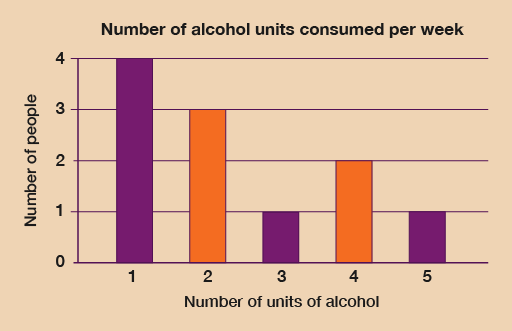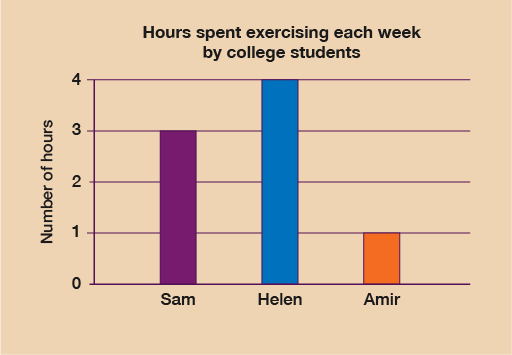Everyday maths for Health and Social Care and Education Support 1

Start this free course now. Just create an account and sign in. Enrol and complete the course for a free statement of participation or digital badge if available.

Free course

# 5 Bar charts

Another way of presenting information would be in a bar chart.

Bar charts are useful because they show data clearly. They must contain the following information:

• A title explaining what the bar chart means.
• Labels that tell you what each bar means. This could be a key or just a label underneath the line that runs along the bottom of the bar graph (the horizontal axis).
• The line going up the left-hand side of the bar graph (the vertical axis) must have numbers at equal intervals. This tells you how big the bars are so that your reader can read the data.

## Example: A healthy lifestyle survey

Let’s have a look at the data from a survey of the number of alcohol units people consume in a week. The data is displayed in the table below:

Number of alcohol units consumed each weekNumber of people
14
23
31
42
5+1

This data could be presented in a bar chart, as follows:Figure 9 Alcohol units bar chart

### Method

Before you start to draw your bar chart, you need to decide what your labels will be and what number intervals you are going to use – that is, how ‘tall’ your bars are going to be.

To do this you need to look at your data and find the biggest number of occurrences (that is, the largest category). In this alcohol units survey this is not too difficult: the most common response was 1 unit of alcohol each week by four people.

This means that the highest number on the vertical axis is 4. The numbers in the survey are all whole numbers so the numbers on this axis will be 0, 1, 2, 3 and 4. The vertical axis should always start at 0 and go up by the same number each time. We can take the label for this axis from the table: ‘Number of people'.

You now need to decide on how many bars you are going to draw. This is already decided for because there are five categories in the survey:

• one unit of alcohol per week
• two units of alcohol per week
• three units of alcohol per week
• four units of alcohol per week
• five units or more of alcohol per week.

So there will be five bars along the horizontal axis of the bar chart, which should be labelled ‘Number of units of alcohol’.

Once you have drawn the axes and labels, you can draw the bars as follows:

• Use a ruler.
• The height of each bar is the number you have for that category.
• The width of the bars must be equal.
• When you have finished drawing your bar chart, don’t forget to give it a title.

### Method summary

• Find out what the highest number of items is. This will give you the biggest number on the vertical axis (the one on the left-hand side). This will be the size of the tallest bar.
• Decide how many bars to draw: this is the number of categories you are dealing with. The bars should be equal in width.
• Draw and label your axes.
• Use a ruler to draw your bars.
• Make up a title for your bar chart.

Now try the following activity. Remember to check your answers once you have completed the questions.

## Activity 9: Creating a bar chart

Three college students were asked how many hours they exercise for each week. The table below shows their responses:

NameWeekly hours of exercise
Sam3
Helen4
Amir1

Draw a bar chart to display this data. Remember to label your axis and give your chart a title.

Check with the following suggestions before continuing;

The highest number of hours is four, so you must ensure that the vertical axis should start at 0 and go up to 4.

You must label the horizontal axis with the names of the students and the vertical axis with the number of weekly hours of exercise.

The title must clearly state what data the bar chart is showing.

Your graph should look something like the following:Figure 10 Weekly hours of exercise bar chart

## Summary

In this section you have learned about how to present data in bar charts.

FSM_SSH_1